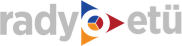HABERİ PAYLAŞIN

TOBB ETÜ Matematik Bölümü yüksek lisans ve doktora öğrencilerimiz, uluslararası hakemli dergilerde yayımlanan makaleleri ile hem Üniversitemizin hem de Ülkemizin uluslararası platformdaki saygınlığını artırma yönünde önemli bir katkı sunmaktadırlar. Bu yayınların büyük bir çoğunluğu “Science Citation Index” tarafından taranan dergilerde bulunmaktadır. Matematik Anabilim Dalındaki tüm lisansüstü öğrencilerimizi kutlar başarılarının devamını dileriz.

Lisansüstü öğrencilerimizin son beş yıldaki TOBB ETÜ adresli bilimsel makaleleri:

• C. Turan and O. Duman, Fundamental properties of statistical convergence and lacunary statistical convergence on time scales, Filomat, 2016 (accepted for publication).
• T. Y. Gökçer and O. Duman, Summation process by max-product operators, in "Computational Analysis", pp. 59-67, Springer Proc. Math. Stat., vol. 155, Springer, New York, 2016.
• İ. Aslan and O. Duman, Summability process on the Baskakov-type approximation, Periodica Math. Hungar. 72 (2016), no. 2, 186-199.
• Ö. Akın, T. Khaniyev, S. Bayeg and İ. B. Turkşen, Solving a second order fuzzy initial value problem using the heaviside function, Turkish Journal of Mathematics and Computer Science 4 (2016), 16-25.
• E. Karaoglu, E. Yılmaz and H. Merdan, Hopf bifurcation analysis of ˘ coupled two-neuron system with discrete and distributed delays, Nonlinear Dynamics, 85 (20016), 1039-1051.
• E. Karaoglu, E. Yılmaz and H. Merdan, Stability and bifurcation analysis of two-neuron network with discrete and distributed delays, Neurocomputing, 182 (2016), 102-110.
• N. Küçük and O. Duman, Summability methods in weighted approximation to derivatives of functions, Serdica Math. J. 41 (2015), 355-368.
• H. Merdan and Ş. Kayan, Hopf bifurctions in Lengyel-Epstein reaction-diffusion model with discrete time delay, Nonlinear Dynamics, 79 (2015), 1757-1770.
• E. Karaoglu and H. Merdan, Hopf bifurcations of a ratio-dependent predator-prey model involving two discrete maturation time delays, Chaos, Solitons & Fractals, 68 (2014), 159-168.
• E. Karaoglu and H. Merdan, Hopf bifurcation analysis for a ˘ ratio-dependent predator-prey system involving two delays, ANZIAM Journal, 55 (2014), 214-231,
• C. Turan and O. Duman, Convergence methods on time scales, AIP Conf. Proc. 1558 (2013) 1120-1123.
• C. Turan and O. Duman, Statistical convergence on time scales and its characterizations, in "Advances in Applied Mathematics and Approximation Theory", pp. 57-71, Springer Proc. Math. Stat., vol. 41, Springer, New York, 2013.
• A. A. Ergür and O. Duman, Generalization of statistical Korovkin theorems, J. Appl. Math. 2013, Article ID 187530, 5 pp.
• Ö. Akın, T. Khaniyev, Ö. Oruç and İ. B. Türkşen, Order fuzzy initial value problems, Expert Systems with Applications, 40 (2013), 953-957.
• H. Akkocaoğlu, H. Merdan and C. Çelik, Hopf bifurcation analysis of a general non-linear differential equation with delay, J. Comput. Appl. Math. 237 (2013), 565-575.
• E. Kılıç and T. Arıkan, More on the infinite sum of reciprocal Fibonacci, Pell and higher order recurrences, Appl. Math. Comput. 219 (2013), 7783-7788.
• O. Duman and M. Kester, Statistical approximation by double Poisson-Cauchy singular integral operators, Results Math. 62 (2012) 53-65.
• Ö. Akın and Ö. Oruç, A prey predator model with fuzzy initial values, Hacettepe J. Math. Stat. 41 (2002), 387-395.
• H. Merdan and E. Karaoğlu, Impact of Allee effects on stability analysis of the population model Xt+1 =aXt f(Xt-3), Hacettepe J. Math. Stat. 41 (2012), Issue: 5, 751-765.
• H. Merdan and H. Çakmak, Liquidity effect on the asset price forecasting, J. Nonlinear Syst. Appl. 3 (2012), 82-87.
• H. Merdan and M. Alişen, A mathematical model for asset pricing, Appl. Math. Comput. 218 (2011), 1449-1456.ŞİMDİ ÇALIYOR